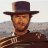# Percent Range Flags Turning Points for thinkorSwim#### Townsend

##### Active member
VIP
This indicator flags areas of low activity. In this example, the percent difference between each 5 bar Length is compared with a PctBelow value. This is a VERY short term chart, so I'm looking any 5 bars that move less than 0.5%. If you're going to try this on longer term charts, you'll need to increase that percentage of the PctBelow value.

In this example, the white label is showing 0.5 percent range for the past 5 bars. It would need to fall just below the 0.5 PctBelow value for a highlight to show on the current bar.

Also, there is a PctAbove value which highlights moves above the PctAbove value.

And... a Pct plot is included, so this can be used in a Scan.Code:
``````# 2019 Paul Townsend

input length = 5;
input PctBelow = 0.5;
input PctAbove = 0.9;

def highLine = Highest(high, length);
def lowLine = Lowest(low, length);
plot Pct =  Round((highLine - lowLine) / lowLine * 100, 1);
pct.hide();
AddLabel(yes, "Pct= " + Pct, Color.WHITE);
#AddLabel(yes, "HL=" + highLine + " LL=" + lowLine + " Pct= " + Pct, Color.WHITE);

plot BoxHigh = if AbsValue(Pct) < PctBelow or AbsValue(Pct) > PctAbove
then highLine else Double.NaN;
plot BoxLow = if AbsValue(Pct) < PctBelow or AbsValue(Pct) > PctAbove
then lowLine else Double.NaN;
boxhigh.hide();
boxlow.hide();

AddCloud(if AbsValue(Pct) < PctBelow then BoxHigh else Double.NaN, BoxLow, Color.yellow, Color.white);
AddCloud(if AbsValue(Pct) > PctAbove then BoxHigh else Double.NaN, BoxLow, Color.white,Color.white);``````#### BenTen

Thanks for sharing this one. Will take it for a spin tonightScan for stocks by their daily percent price range? Questions 3